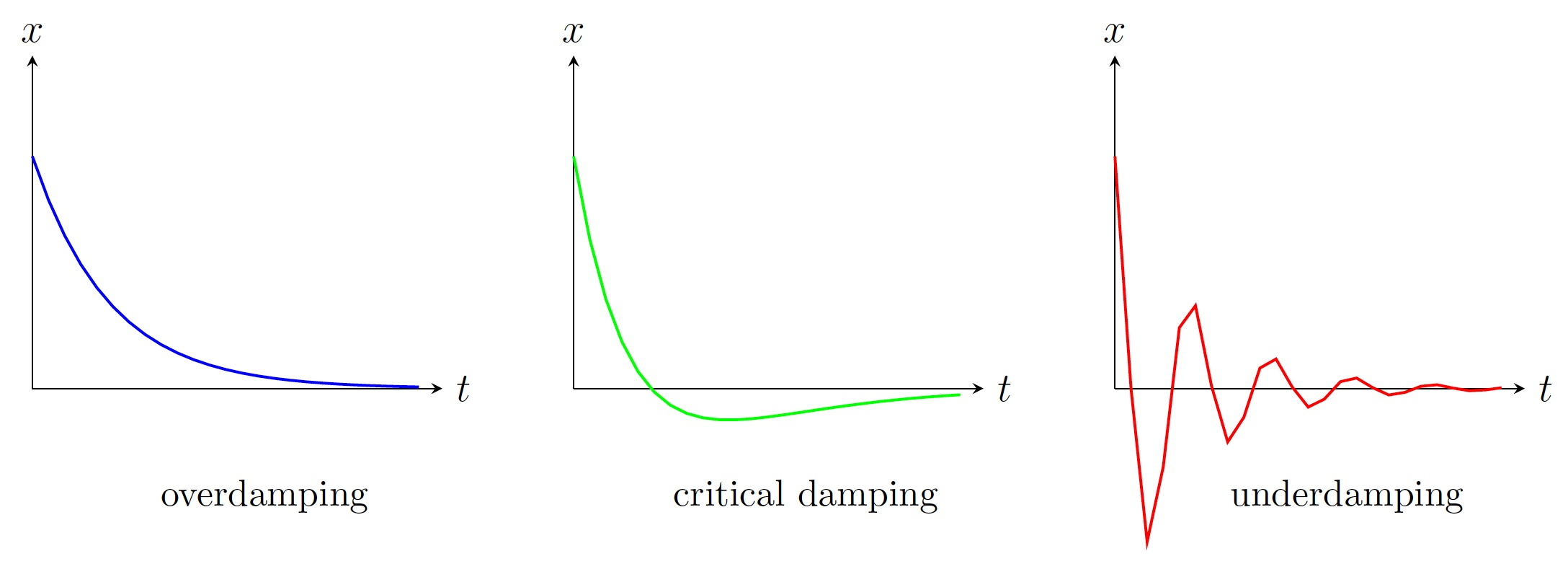DiffEq Home

## Modeling with Second Order ODEs

Motion of a simple pendulum:
Consider a "simple'' pendulum with length $$L$$. Let $$\theta(t)$$ be its angular displacement at time $$t$$. By Newton's second law (i.e., mass $$\cdot$$ acceleration= net force) we get \begin{align*} &\frac{d^2\theta}{dt^2}=-\frac{g}{L}\sin \theta\\ \text{i.e., }&\frac{d^2\theta}{dt^2}+\frac{g}{L}\sin \theta =0. \end{align*} This nonlinear ODE has no solution in terms of usual functions. So it is linearized by taking $$\sin \theta\approx \theta$$ for $$\theta\approx 0$$: $\frac{d^2\theta}{dt^2}+\frac{g}{L} \theta =0.$ The characteristic equation is $r^2+\frac{g}{L}=0 \implies r=\pm i\sqrt{\frac{g}{L}}.$ The general solution is $\theta=c_1\cos\left(\sqrt{\frac{g}{L}}t \right)+c_2\sin\left(\sqrt{\frac{g}{L}}t \right).$ Using the initial conditions $$\theta(0)=\theta_0$$ and $$\theta'(0)=0$$, we get $$c_1=\theta_0$$ and $$c_2=0$$. Thus $\theta=\theta_0\cos\left(\sqrt{\frac{g}{L}}t \right).$

Kirchhoff's voltage law:
Consider an electric circuit with a resistor, an inductor, a capacitor, and a battery arranged in series (RLC circuit). Let $$Q(t)$$ be the charge of the capacitor and $$I(t)$$ current at time $$t$$. Then Kirchhoff's voltage law states that the sum of the voltage drops across the resistor, inductor, and capacitor is equal to the supplied voltage $$V(t)$$: $L\frac{dI}{dt}+RI+\frac{Q}{C}=V,$ where $$L$$ is the constant inductance, $$R$$ is the constant resistant and $$C$$ is the constant capacitance. Since $$I$$ is the rate of change of $$Q$$ with respect to time $$t$$, $$I=\frac{dQ}{dt}$$. Then the preceding ODE becomes $L\frac{d^2Q}{dt^2}+R\frac{dQ}{dt}+\frac{Q}{C}=V.$

Vibrating springs:
Consider a horizontal or a vertical spring with one end attached to a firm base and the other end an object of mass $$m$$. If the spring is stretched or compresses $$x$$ units from its equilibrium position, it exerts a restoring force $$F_r$$ which is proportional to $$x$$ by Hooke's Law: $F_r=-kx,$ where $$k > 0$$ is the spring constant. Ignoring other forces like friction, by Newton's Second Law we get $m\frac{d^2x}{dt^2}= F_r \implies m\frac{d^2x}{dt^2}=-kx \implies m\frac{d^2x}{dt^2}+kx=0.$ The characteristic equation is $$mr^2+k=0\implies r=\pm i\sqrt{k/m}$$. So the general solution is $x=c_1\cos(\sqrt{k/m} t)+c_2\sin(\sqrt{k/m} t)=A\cos(\omega t+\delta),$ where $$A=\sqrt{c_1^2+c_2^2}$$, $$\omega=\sqrt{k/m}$$, and $$\delta=\tan^{-1}(c_2/c_1)$$ are the amplitude, frequency, and phase angle respectively. This is an example of simple harmonic motion.

Damped vibrations:
Consider a horizontal spring with friction of the surface or a vertical spring with a damping force of the medium. The damping force $$F_d$$ is modeled to be proportional to the velocity, i.e., $F_d=-c\frac{dx}{dt},$ where $$c > 0$$ is the damping constant. By Newton's Second Law we get $m\frac{d^2x}{dt^2}= F_r+F_d \implies m\frac{d^2x}{dt^2}=-kx-c\frac{dx}{dt} \implies m\frac{d^2x}{dt^2}+c\frac{dx}{dt}+kx=0.$ The characteristic equation is $$mr^2+cr+k=0\implies r_1,r_2=(-c\pm \sqrt{c^2-4mk})/2m$$.

1. $$c^2-4mk > 0$$ (overdamping): Roots are distinct real negative and $$x=c_1e^{r_1t}+c_2e^{r_2t}$$. Here $$c > 2\sqrt{mk}$$ implies a high damping force resulting in no vibration.

2. $$c^2-4mk=0$$ (critical damping): $$r_1=r_2=-c/2m$$ and $$x=(c_1+c_2t)e^{-ct/2m}$$. Here $$c=2\sqrt{mk}$$ implies the least damping force that suppresses too much vibrations.

3. $$c^2-4mk < 0$$ (underdamping): Roots are complex conjugates and $$x=e^{-ct/2m}(c_1\cos(\omega t)+c_2\sin(\omega t))$$, $$\omega=\sqrt{4mk-c^2}/2m$$. Here $$c < 2\sqrt{mk}$$ implies a low damping force failing to suppress too much vibrations.Last edited